Courses

# Ionic Equilibrium 03-01-2019

## 30 Questions MCQ Test AAKSC Chemistry Mock test Series | Ionic Equilibrium 03-01-2019

Description
This mock test of Ionic Equilibrium 03-01-2019 for Class 12 helps you for every Class 12 entrance exam. This contains 30 Multiple Choice Questions for Class 12 Ionic Equilibrium 03-01-2019 (mcq) to study with solutions a complete question bank. The solved questions answers in this Ionic Equilibrium 03-01-2019 quiz give you a good mix of easy questions and tough questions. Class 12 students definitely take this Ionic Equilibrium 03-01-2019 exercise for a better result in the exam. You can find other Ionic Equilibrium 03-01-2019 extra questions, long questions & short questions for Class 12 on EduRev as well by searching above.
QUESTION: 1

### For which salt  the pH of its solution does not change with dilution?

Solution:

pH of salt of weak acid and weak base is drived by the relation :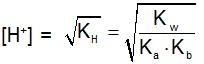QUESTION: 2

### Which is the strongest acid?

Solution:

Trichloroacetic acid is almost of same strength as HCI due to indictive effect of CI-atoms.

QUESTION: 3

### The conjugate base of [AI(H2O)3(OH)3] is:

Solution:

Conceptual

QUESTION: 4

Which equilibrium can be decribed as Lewis acid-base reaction but not Bronsted acid-base reaction?

Solution:

It involves gain and loss of electron pair(Lewis concept)

QUESTION: 5

The correct representation for the solubility product constant of Ag2CrO4 is:

Solution:
QUESTION: 6

The correct statement for the equilibrium is:
HCIO4 + H2O  ⇌ H3O+ + CIO-4

Solution:

Both CIO-4 (base) and HCIO4 (acid) differ by a proton and thus, are conjugate pairs of acid and base.

QUESTION: 7

The rapid change of pH near the stochiometric point of an acid-base titration is the basic of indicator detection. pH of the solution is related to the ratio of the  concentration of conjugate acid (HIn) and base (In- ) form of the indicator by the expression:

Solution: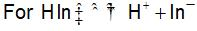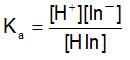∴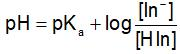QUESTION: 8

One litre of water contains 10-7 mole H+ ions. Degree of ionisation of water is:

Solution: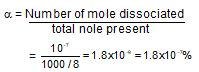Total mole of H2O in 1 litre

QUESTION: 9

If  CuSO4 + 5H2O (s)  ⇌ CuSO4 . 3H2O(S) + 2H2O(v)Kp = 1.086 * 10-4  atm2 at 250C. The efflorescent nature of  CuSO5H2O can be noticed when vapour pressure of H2O in atmosphere is:

Solution:

An efflorescent salt is one that loses H2O to atmosphere. For the reaction,
CuSO4 + 5H2O(s)  ⇌ CuSO4+ 3H2O(s) + 2H2O(v)
Kp = (PH2O)2 = 1.086*10-4
P H2O = 1.04 * 10-2atm = 7.92mm
if P'H2O AT 250C < 7.92 mm only then, reaction will proceed in forward direction.

QUESTION: 10

The equilibrium constant for the reaction, 3C2H2  ⇌ C6H,is 4.0 at T.K. If the equilibrium concentration of C2H2 is 0.5 mol/litre, the concentration of C6H6 is:

Solution: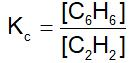or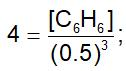∴  [C6H6]=0.5 M

QUESTION: 11

40% of a mixture of 0.2 mole of N2 and 0.6 mole of H2 react to give NH3 according to the equation, N2(g) + 3h2(g)  ⇌2NH3 (g) at constant temperature and pressure. Then the ratio of the final volume to the initial volume of gases is:

Solution: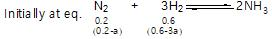Total mixture is 0.8;40% of it reacts, i.e,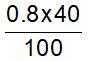reacts to give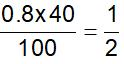mole of NH3
or    2a=0.16

∴    a=0.08
∴   Initial mole=0.8
Final mole = (0.2-0.08)+(0.6-0.24)+0.16
= 0.12+0.36+0.16=0.64
Ratio of final to initial mole = 0.64/0.8 = 0.8 = 4/5

QUESTION: 12

When 20 g of CaCO3 were put into 10 litre flask and heated to 8000C, 35% of CaCO3 remained unreacted at equilibrium. Kp for decomposition of CaCO3 is:

Solution:

CaCO3(s)  ⇌ CaO(s) + CO2(g)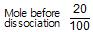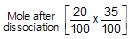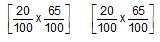Mole of CO2 formed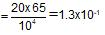PV = nRT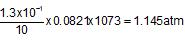Now,    Kp = Pco2 = 1.145 atm

QUESTION: 13

The value of Kp1 and Kp2 for the reactions
X  ⇌ Y+Z ..(1)
A  ⇌ 2b ....(2)
are in the ration 9 : 1. If degree of dissociation of X and A be equal, them total pressure at equilibrium (1) and (2) are in the ratio:

Solution: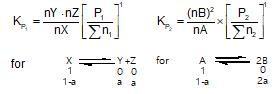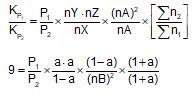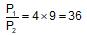QUESTION: 14

At constant temperature in one litre vessel, when the reaction, 2SO3(g)→2SO2(g)+O2(g) is at equilibrium, the SOconcentration is 0.6 M, initial concentration of SO3 is 1 M. The equilibrium constant is:

Solution: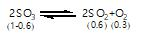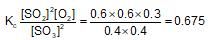QUESTION: 15

HCOOH  ⇌ HCOO- + H+; Ks = 1.7*10-4
The ionization of formic acid is represented above. Calculate [H+] of a solution initially containing 0.1 M HCOOH and 0.05 M HCOONa:

Solution: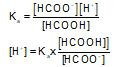= 3 * 10-4

QUESTION: 16

When NaCI is added gradually to the saturated solution of AgCI then which of the following plot is correct?

Solution:

Conceptual

QUESTION: 17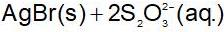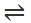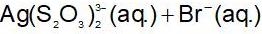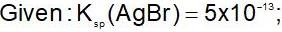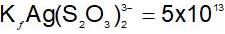What is the molar solubility of AgBr in 0.1 M Na2S2O3?

Solution:

AgBr(s) ⇌ Ag+(aq) + Br- (aq) Ksp

Ag+(aq)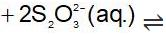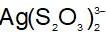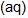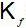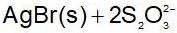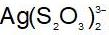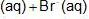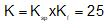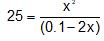x = 0.045M

QUESTION: 18

The solubility product of different sparingly soluble salts are:
(i) XY = 4x10-20
(ii) X2Y = 3.2x10-11
(iii)  XY= 2.7 x 10-31
The increasing order of solubility is:

Solution: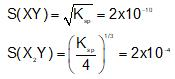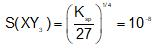QUESTION: 19

An aqueous solution of hydrogen sulphide shows the equilibrium,
H2S ⇌ H+ +HS-
If dilute hydrochloric acid is added to an aqueous solution of hydrogen sulphide without any change in temperature, then:

Solution: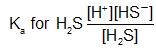An increase in [H+] will show a decrease in [HS- ] to maintain constant Kavalue.

QUESTION: 20

The equilibrium constant for decomosition of Hbr at 500 K and 700 K are 10-10 and 10-5 respectively. The reaction is:

Solution: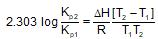Thus, if Kp2 > Kp1;T2 > T1,then = +ve

QUESTION: 21

The reaction quotient (Q) for the reaction is given by:
N2(g)+3H2(g) ⇌ 2NH3;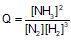The reaction will proceed from right to left if:

Solution:

Q > Kc thus, Q must decrease so that [NH3] may decrease to have backward reaction.

QUESTION: 22

Which of the following gas phase reaction has Kp less than Kc?

Solution:

Δn= -1 and Kp = Kc x (RT)Δn

QUESTION: 23

For the gaseous phase reaction, 2NO ⇌ N2 + O2, ΔH° = -43.5 kcal mol-1 which statement is correct for,
N2(g)+O2(g) ⇌ 2NO(g) ?

Solution: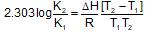ΔH= +ve for the reaction,
N2 + O2 ⇌ 2NO

QUESTION: 24

The volume of the reactions vessel containing an equilibrium mixture in the reaction.
SO2Cl2 (g) ⇌ SO2(g)+Cl2(g)
is increased. When equilibrium is restablished.

Solution:

An increase in volume for the equilibrium having,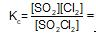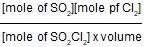will increase the mole of CI2 or SO2 to maintain Kc values constant

QUESTION: 25

100ml of 1N NH4OH (Kb=5x10-5) is neutralized to equivalence point by 1N HCl. The PH of solution at equivalence point is

Solution:

Meq. of NH4OH = Meq. of HCI
= Meq. of NH4CI formed
100 x 1=1xV
= Meq. of NH4CI
Also,    V = 100 mL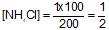Due to hydrolysis of NH4CI;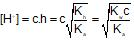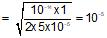∴ pH = 5

QUESTION: 26

Number of H+ ions present in 10 mL of a solution of pH =  3 is:

Solution:

Meq. of H+ = 10-3 x 102
∴  No. of H+ =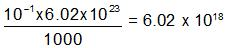QUESTION: 27

To 100 mL of 0.1 M AgNO3 solution solid K2SO4 is added. The concentration of K2SO4 that shows the precipitation is,(Ksp for Ag2SO4 = 6.4 x 10-5 M)

Solution:

[Ag+]2[SO2-4]=Ksp
∴ [SO2-4] =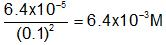QUESTION: 28

The composition of an acidic buffer mixture made up of HA and NaA of total molarity 0.29 having pH = 4.4 and Ka = 1.8 x 10-5 in terms of concentration of salt and acid respectively is:

Solution:

pH = -logKa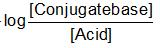Let a mol litre-1 be concentration of salt; then concentration of acid = (0.29-a)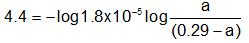∴ a=0.09
∴ Conjugate base = [Salt] = 0.09 M
[Acid] = 0.29 - 0.09 = 0.20 M

QUESTION: 29

At 250 C, Kb for a base BOH is 1.0 x 10-12. The [OH- ] in 0.01 M aqueous solution of base is:

Solution: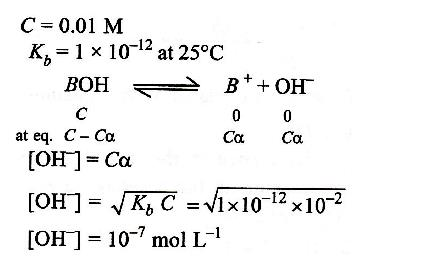QUESTION: 30

Which of the following is a buffer solution?

Solution:

Buffer solution will conatin the mixture of CH3COOH and CH3COONa